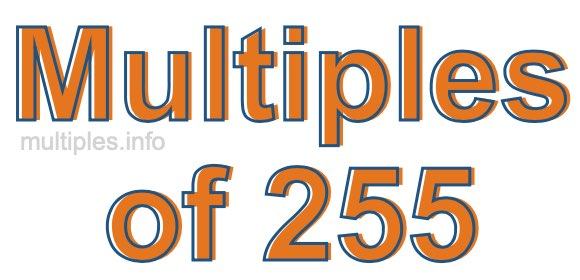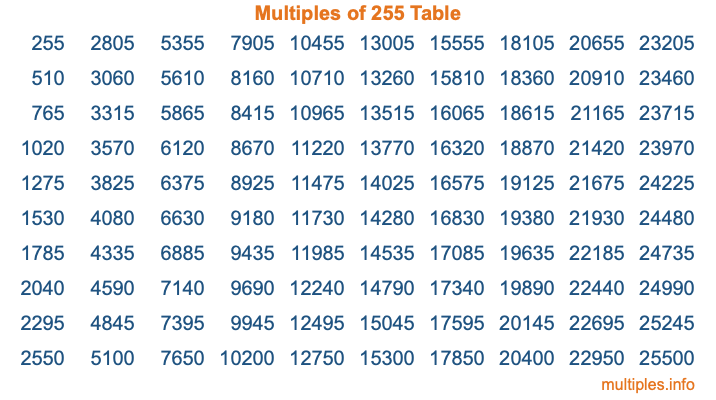Multiples of 255Welcome to the Multiples of 255 page. Here we will first teach you everything you will ever need to know about the multiples of 255, and then give you a study guide summary of everything we taught you to make sure you remember it all. Use this page to look up facts and learn information about the multiples of 255. This page will make you a multiples of two hundred fifty-five expert!

Definition of Multiples of 255
Multiples of 255 are all the numbers that when divided by 255 equal an integer. Each of the multiples of 255 are called a multiple. A multiple of 255 is created by multiplying 255 by an integer.

Therefore, to create a list of multiples of 255, you start with 1 multiplied by 255, then 2 multiplied by 255, then 3 multiplied by 255, and so on for as long as you want. Thus, the list of the first five multiples of 255 is 255, 510, 765, 1020, and 1275. To see a larger list of multiples of 255, see the printable image of Multiples of 255 further down on this page. We also have a category where you can choose any nth multiple of 255.

Multiples of 255 Checker
The Multiples of 255 Checker below checks to see if any number of your choice is a multiple of 255. In other words, it checks to see if there is any number (integer) that when multiplied by 255 will equal your number. To do that, we divide your number by 255. If the the quotient is an integer, then your number is a multiple of 255.

Is  a multiple of 255?

Least Common Multiple of 255 and ...
A Least Common Multiple (LCM) is the lowest multiple that two or more numbers have in common. This is also called the smallest common multiple or lowest common multiple and is useful to know when you are adding our subtracting fractions. Enter one or more numbers below (255 is already entered) to find the LCM.

Check out our LCM Calculator if you need more details about the Least Common Multiple or if you need the LCM for different numbers for adding and subtraction fractions.

nth Multiple of 255
As we stated above, 255 is the first multiple of 255, 510 is the second multiple of 255, 765 is the third multiple of 255, and so on. Enter a number below to find the nth multiple of 255.

th multiple of 255

Multiples of 255 vs Factors of 255
255 is a multiple of 255 and a factor of 255, but that is where the similarities end. All postive multiples of 255 are 255 or greater than 255. All positive factors of 255 are 255 or less than 255.

Below is the beginning list of multiples of 255 and the factors of 255 so you can compare:

Multiples of 255: 255, 510, 765, 1020, 1275, etc.

Factors of 255: 1, 3, 5, 15, 17, 51, 85, 255

As you can see, the multiples of 255 are all the numbers that you can divide by 255 to get a whole number. The factors of 255, on the other hand, are all the whole numbers that you can multiply by another whole number to get 255.

It's also interesting to note that if a number (x) is a factor of 255, then 255 will also be a multiple of that number (x).

Multiples of 255 vs Divisors of 255
The divisors of 255 are all the integers that 255 can be divided by evenly. Below is a list of the divisors of 255.

Divisors of 255: 1, 3, 5, 15, 17, 51, 85, 255

The interesting thing to note here is that if you take any multiple of 255 and divide it by a divisor of 255, you will see that the quotient is an integer.

Multiples of 255 Table
Below is an image of the first 100 multiples of 255 in a table. The table is in chronological order, column by column. The first column has the first ten multiples of 255, the second column has the next ten multiples of 255, and so on.The Multiples of 255 Table is also referred to as the 255 Times Table or Times Table of 255. You are welcome to print out our table for your studies.

Negative Multiples of 255
Although not often discussed or needed in math, it is worth mentioning that you can make a list of negative multiples of 255 by multiplying 255 by -1, then by -2, then by -3, and so on, to get the following list of negative multiples of 255:

-255, -510, -765, -1020, -1275, etc.

Multiples of 255 Summary
Below is a summary of important Multiples of 255 facts that we have discussed on this page. To retain the knowledge on this page, we recommend that you read through the summary and explain to yourself or a study partner why they hold true.

There are an infinite number of multiples of 255.

A multiple of 255 divided by 255 will equal a whole number.

255 divided by a factor of 255 equals a divisor of 255.

The nth multiple of 255 is n times 255.

The largest factor of 255 is equal to the first positive multiple of 255.

255 is a multiple of every factor of 255.

255 is a multiple of 255.

A multiple of 255 divided by a divisor of 255 equals an integer.

255 divided by a divisor of 255 equals a factor of 255.

Any integer times 255 will equal a multiple of 255.

Multiples of a Number
Here you can get the multiples of another number, all with the same attention to detail as we did for multiples of 255 on this page.

Multiples of
Multiples of 256
Did you find our page about multiples of two hundred fifty-five educational? Do you want more knowledge? Check out the multiples of the next number on our list!